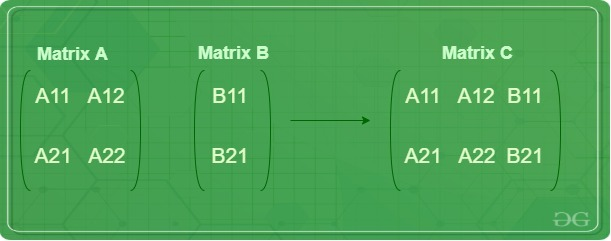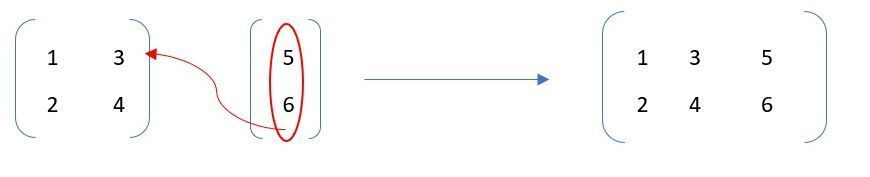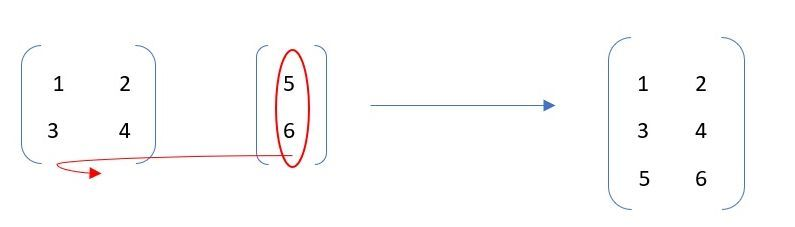# Combining Matrices in R

Combining matrices involves the concatenation of two or more smaller matrices, either row or column wise to form a larger matrix. It is basically a data manipulation operation where the involved matrices must be of compatible sizes to execute the operation. Matrices can be combined either horizontally or vertically.There are two ways of combining matrices in R:

• Column-wise combination
• Row-wise Combination

#### Column-Wise Combination

Column bind, cbind() function in R, is used to merge two data frames or matrices(n may or may not be equal to p) together by their columns. The matrices involved should have the same number of rows.Example:

 `# R program for combining two matrices ` `# coulumn-wise ` ` `  `# Creating 1st Matrix ` `B ``=` `matrix(c(``1``, ``2``), nrow ``=` `1``, ncol ``=` `2``)  ` ` `  `# Creating 2nd Matrix ` `C ``=` `matrix(c(``3``, ``4``, ``5``), nrow ``=` `1``, ncol ``=` `3``) ` ` `  `# Original Matrices ` `print``(B) ` `print``(C) ` ` `  `# Combining matrices ` `print` `(cbind(B, C)) `

Output:

```     [,1] [,2]
[1,]    1    2
[,1] [,2] [,3]
[1,]    3    4    5
[,1] [,2] [,3] [,4] [,5]
[1,]    1    2    3    4    5
```

In the above code, the columns [3 4 5] of matrix C are added in order to the columns [1 2] of the matrix B. These changes are not made to any of the existing matrices.

Properties:

• The number of columns of the resultant matrix is the sum of the columns of the two matrices.
• Non-Commutative: The columns are merged in the order in which the the parameters are specified in the function. Therefore, cbind(a, b) != cbind(b, a)
• Associative: cbind(cbind(a, b), c) = cbind(a, cbind(b, c))

#### Row-Wise Combination

Row bind, rbind() function in R, is used to merge two data frames or matrices(m may or may not be equal to n), together by their rows. The matrices involved should have the same number of columns.Example:

 `# R program for combining two matrices ` `# row-wise ` ` `  `# Creating 1st Matrix ` `B ``=` `matrix(c(``1``, ``2``, ``3``), nrow ``=` `1``, ncol ``=` `3``) ` ` `  `# Creating 2nd Matrix ` `C ``=` `matrix(c(``4``, ``5``, ``6``, ``7``, ``8``, ``9``), nrow ``=` `2``, ncol ``=` `3``) ` ` `  `# Original Matrices ` `print``(B) ` `print``(C) ` ` `  `# Combining matrices ` `print` `(rbind(B, C)) `

Output:

```     [,1] [,2] [,3]
[1,]    1    2    3
[,1] [,2] [,3]
[1,]    4    6    8
[2,]    5    7    9
[,1] [,2] [,3]
[1,]    1    2    3
[2,]    4    6    8
[3,]    5    7    9
```

The rows [4 6 8] and [5 7 9] are appended to a row of matrix B[1 2 3] in order. No changes are made to the original matrices.

Properties:

• The number of rows of the resultant matrix is the sum of the rows of the two matrices.
• Non-Commutative: The rows are merged in the order in which the the parameters are specified in the function. Therefore, rbind(a, b) != rbind(b, a)
• Associative: rbind(rbind(a, b), c) = rbind(a, rbind(b, c))

Time Complexity: O(m+n)
Space Complexity: O(m+n), where m is the total number of elements of first matrix and n of the second matrix.

My Personal Notes arrow_drop_upCheck out this Author's contributed articles.

If you like GeeksforGeeks and would like to contribute, you can also write an article using contribute.geeksforgeeks.org or mail your article to contribute@geeksforgeeks.org. See your article appearing on the GeeksforGeeks main page and help other Geeks.

Please Improve this article if you find anything incorrect by clicking on the "Improve Article" button below.

Article Tags :

1

Please write to us at contribute@geeksforgeeks.org to report any issue with the above content.